# ISEE Upper Level Math : How to factor a number

## Example Questions

2 Next →

### Example Question #21 : Numbers And Operations

How many integers from 51 to 70 inclusive do not have 2, 3, or 5 as a factor?

NoneExplanation:

We can eliminate the ten even integers right off the bat, since, by definition, all haveas a factor. Of the remaining (odd) integers, we eliminateand, as they haveas a factor. What remains is: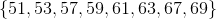We can now eliminate the multiples of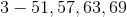. This leaves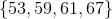.

The correct choice is.

### Example Question #22 : Numbers And Operations

Add the factors of.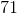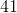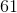Explanation:

The factors ofare: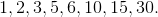Their sum is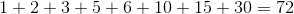.

### Example Question #23 : Numbers And Operations

Factor the number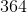to all of its prime factors.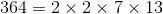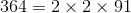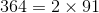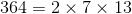Explanation:

Use a tree to find all of the factors of.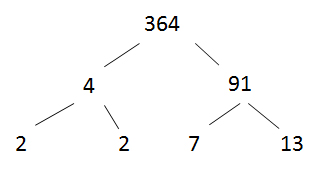The prime factors ofare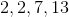.

### Example Question #11 : How To Factor A Number

Adam fills up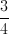of his glass in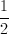of a minute.  What is the total time, in seconds, that it takes him to fill up his entire glass?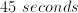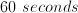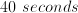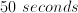Explanation:

There are more than one ways to go about solving this problem.

The easiest was probably involves converting theminute to 30 seconds as soon as possible.

Now we can see that Adam has filledof his cup in 30 seconds.  We can also see that he needs to fill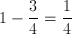of his cup to fill his cup entirely.  Since 3 of those quarters fill up in 30 seconds, then 1 of those quarters can be filled in 10 seconds  Thus Adam needs an additional 10 seconds to finish filling his glass, or a total of 40 seconds.

### Example Question #25 : Numbers And Operations

Find the prime factorization for 72.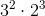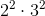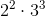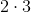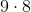Explanation:

To find the prime factorization, start by breaking 72 down. I picked, which can be broken down further to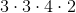. The 4 can be broken down further, so go one more step to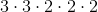. The answers are given in exponents so give your answer in that format: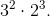### Example Question #21 : Numbers And Operations

What are all of the prime factors of 34?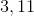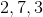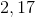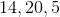Explanation:

What are all of the prime factors of 34?

We need to find which prime numbers can be multiplied to get to 34.

We can find these numbers by dividing prime numbers out one at a time.

Recall that a prime factor is a number which is only divisible by one and itself.

When performing prime factorization on an even number, always begin by pulling out 2.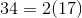Now, we are essentially done, because 17 is also a prime number. So, the prime factors of 34 are 2 and 17.

### Example Question #27 : Numbers And Operations

What is the prime factorization of 78?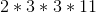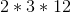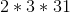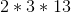Explanation:

What is the prime factorization of 78?

To find the prime factorization of a number, we need to find all the prime numbers which, when multiplied, give us our original number.

When starting with an even number, find the PF by first pulling out a two.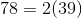Next, what can we pull out of the 39? Let's try 3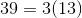Can we pull anything out of the 13? Nope!